This video was brought to you by GeneralPAC.com, making power systems Intuitive, Open and Free for Everyone, Everywhere. Consider subscribing and supporting through patreon.com/GeneralPAC. This is a mechanism for you to support us financially so we can continue making high quality power system video tutorials. Our corporate sponsor for this topic is AllumiaX.com from Seattle, Washington. Contact them for industrial and commercial power system studies.

Introduction to the delta WYE Transformer connection, part 7e.

In this part we're going to continue our discussion about the clock system that is used to describe the phase relationship between the delta WYE Transformer connection and other Transformer connections as well.

So let's get started, so in the previous videos, we drew this diagram and really understood all of the pieces and how they all work together.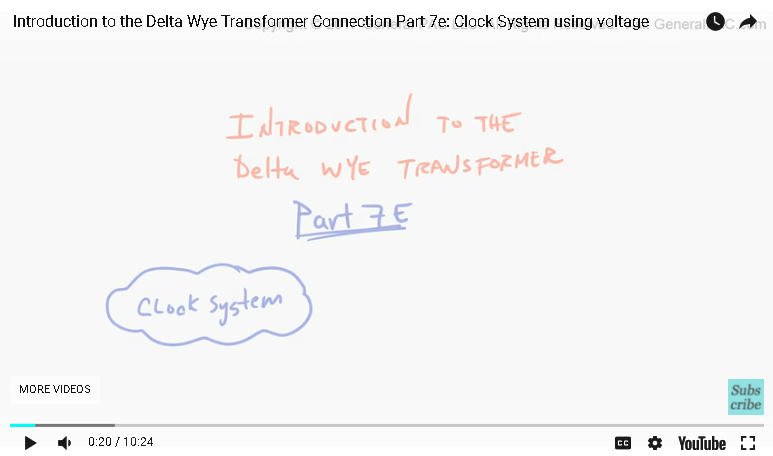What we concluded was the Vag on the high voltage side that is used as a reference quantity and what I'm comparing that to this Vag here that is also used as a reference so these two pieces of information is critical and what we ended up doing was drawing a phasor diagrams so we gonna draw that very quickly right here, we said that if Vag, Vbg and VCG in a balanced system such that the phasors rotated in the counter clockwise direction and were in a ABC phase sequence, then this Transformer connection which we know as the DAC and we are claiming as a DYN11 this Transformer connection would produce a resultant vector.

Now before we draw the resultant vector, we should really think about what it is that we really want to relate this reference voltage with this reference voltage, so to connect the dots, first we're going to perform this equation which we have done multiple times in the past but I'm just gonna do one more time here so we know the Vag - Vbg is equal to Vab right.

We know this, and we have done this multiple times in the past and we know that Vab, because this is a delta transformer connection. We know that the Vab is equal to Vphase A.

Right , really just by inspection Voltage Vab, which is this two points here or the same two points between here and here which is equal to Vphase A, so now we have to refer that back into our phasor diagram, so we perform the phasor diagram using the head to tail method by simply subtracting Vag – Vbg, which is gonna look something like that so just right here is Vbg and our resultant vector which is something like that that is equal to Vab and we know that Vab is equal to Vphase A. The other thing which we have said about the Vphase A, the guy here is that it has the same phase angle as a secondary winding voltage, but different magnitude so that might look like something like.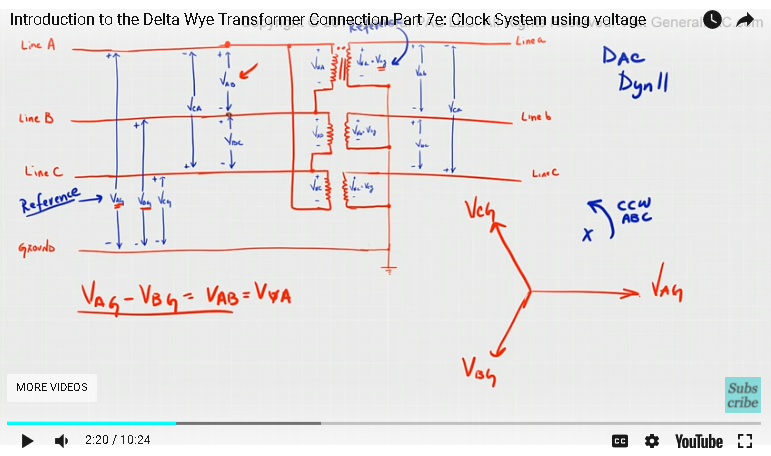So this yellow, we may be able to represent that as Vphase A which is equal to Vag. Vphase A has the same angle as the Vphase A on the primary side but with a different magnitude

Now, what we see here is that we have two voltages, that we can compare these reference voltages with those two voltages or simply this voltage here and this voltage there this is the primary line to ground voltage and this right here is a secondary line to ground volatge that was just reviewed and we talked about this in the previous tutorial, this is how the clock system now works.

So clock system just like a face of a clock has 12 heads right, it has 12 6 3 and 9, it also has 1 2 4 5 7 10 and 11, the way to describe this same relationship using the clock system is is very easy the only thing that we really have to keep in mind is a reference voltages, the reference voltages is referred voltages is Vag on the high voltage side and Vag on the low voltage side, so what we do, we take a high voltage line to ground voltage and we line it up with a 12.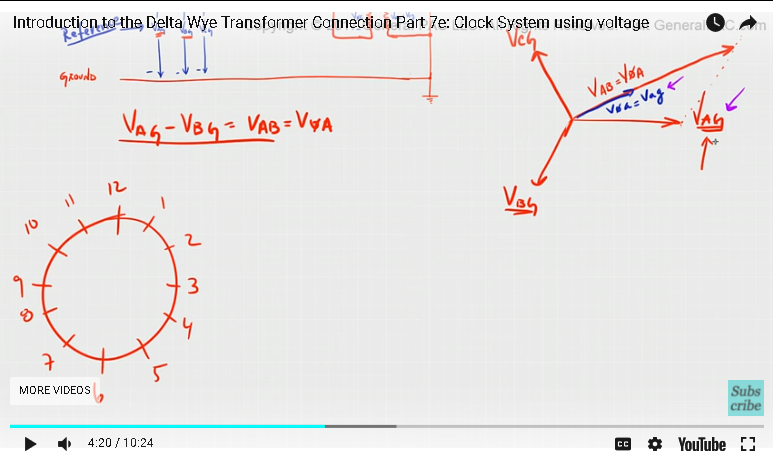Okay, and this is always the HV side first, HV side, the high voltage side is always lined up with the the 12, and then we line up the phasors to see where the secondary line to ground voltage would fall. Okay, so if you just if we just rotate this phasor here, the entire phasor rotated in a counter clockwise direction by 90° if you just take everything rotated by 90 degrees counter clockwise, so either Vphase A or Vag on secondary site is going to fall like that.

Right, so this is Vag on the primary side and this is the Vag on the secondary site so thats how are you set up the clock system using the phasor diagrams now how do we interpret this like if you were given this diagram here what information would be get out of it right, so the way, we interpret this, that we know that the phasors pointing on the 12, we know that it is always the high voltage side.

Okay, and the other phasor that’s pointing on the other number, we know that it is always on the low voltage side. So the way, we interpret this, is that the low voltage reference quantity lags, so it is gonna be lags okay with the high voltage reference quantity by 11 times 30 or 330 degree so this 11 comes from the fact that the low voltage reference quantity is pointing towards the 11 here so that’s pretty clear.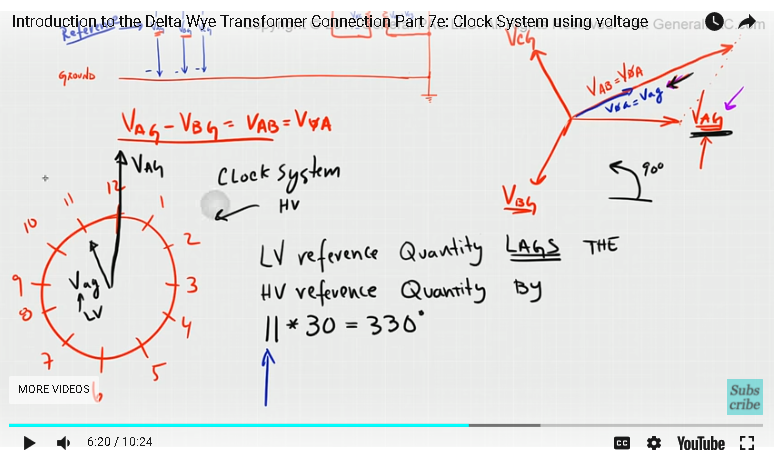So this 30 comes from the fact that in our phasor diagram we always have a 30 degrees increment for the delta connection so every delta connection is gonna have some increment of 30°.

Ok, so the hands of a clock between 12 or 1 that represents 30 degrees between 1 and 2 which represents 30° and if you just count up all the way to 11, is 330 degrees for 30 times 11 or 330 degrees, so it goes back to the same that exactly we are saying in the time, when we were saying that the secondary voltage which is here the secondary voltage, the Yellow Phasor here that lags the primary voltage which is this guy here by 330 degree as if phasors rotate in the counter clockwise direction.

Okay, given that is an ABC phase sequence. Another way of saying that is that the secondary voltage which is Vag again leads the primary voltage by 30 degrees as the phasors rotate in the counter clockwise direction, so this is how we represent the clock system using this particular phasor diagram and using this particular Dac or DYN11 Transformer connection so let's quickly talk about this representation we already know what this Dac means.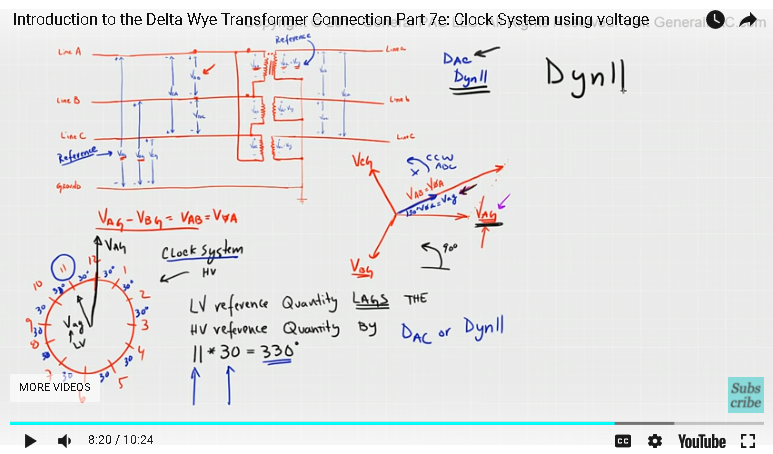So let’s quickly talk about this DYN11 and understand where exactly each term mean, so the D, this D represents that the Transformer connection on the high voltage side that is connected in Delta we don't know how the delta is configured out there, either it's Dac or Vag, we don't know that information but we know that it is connected in Delta, right, the capital D representatives to lower case WYE that represents the low voltage side, and we know that the low voltage side is connected in Wye and that the “n” this here, represents that the low voltage neutral bushing which is this right here, this neutral bushing is brought out which means that the utility or whoever is using this Transformer they can actually grounded or leave it ungrounded, it only represents the fact that the neutral can be grounded if the user choses to, so 11, this 11 represents the low voltage reference quantity lagging the high voltage reference quantity by 11 times 30 or 330 degrees and other way of saying that the low voltage leads the high voltage by 30 degrees okay, so that is how this method of describing a delta WYE Transformer connection is described.

In part 7f, we were used another reference quantity to describe the same exact principles and instead of using line to ground voltage quantities, we will use line current quantity to describe the phase relationship and this method here using line currents are a lot more simpler and lot more sense. Thank you.

#### Greetings from the GeneralPAC Team!

We make high-quality Power Systems Video Tutorials on complex topics that are free and open to everyone!  Thank you so much for supporting us through Patreon so we can continue doing good and valuable work.

What is Patreon and why do we use it?

Patreon is a fantastic portal that allows our fans and community to make monthly contribution (like Netflix subscription) so we can continue creating high-quality power systems video tutorials. In return, you get access to incredible perks like voting on future topics, getting your questions answered, access to VIP Q/A webinars with the creators of GeneralPAC, and much more! We THANK YOU for supporting us

Why do we need your support?

An incredible amount of time and effort is needed to develop high-quality video tutorials. Each video (Part 1 for example) takes approximately 10 hours to complete which includes learning the concept ourselves, brainstorming creative ways to teach and explain the concepts, writing the script, audio recording, video recording, and editing. It's no wonder why Hundreds-of-Thousands of people have watched, liked, subscribed, and left positive comments on Youtube channel. Your support truly makes all the difference.